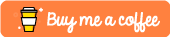# Object Detection with OpenCV and Python

## Introduction

Hello! In this tutorial I will show how to implement simple object detection using Python and OpenCV. 😀

This tutorial requires the weights etc from the below post: https://dev.to/ethand91/setting-up-yolo-with-darknet-1ehm

## Setting up the project

First we need to add the following files into the "weights" directory:

• coco.names
• yolov3.cfg
• yolov3.weights

Also we need to initialize the virtual environment:

``````python3 -m venv env
source env/bin/activate

mkdir weights
cp [darknet directory]/cfg/coco.names weights/
cp [darknet directory]/cfg/yolov3.cfg weights/
cp [darknet directory]/yolov3.weights
``````

## Installing the dependencies

Next we need to install the dependencies needed for the example, create a "requirements.txt" file and add the following:

``````# requirements.txt
opencv-python
argparse
numpy
``````

Then install via:

``````pip install -r requirements.txt
``````

## Writing the source code

First we need to import the needed modules:

``````
import numpy as np
import argparse
import cv2
``````

Next declare the necessary variables and initialize the network model:

``````LABELS_FILE = "weights/coco.names"
CONFIG_FILE = "weights/yolov3.cfg"
WEIGHTS_FILE = "weights/yolov3.weights"
CONFIDENCE_THRESHOLD = 0.3

np.random.seed(4)
COLORS = np.random.randint(0, 255, size = (len(LABELS), 3), dtype = "uint8")

``````

The following function loops through the detected objects found in the image, checks to see if the confidence is above the minimal threshold and if so adds the box into the boxes array along with the coordinates the detection was discovered.

It then checks to make sure there is more than one detection and if so it draws the box along with the object label and confidence onto the image.

Finally the modified image is then shown on screen.

``````def drawBoxes (image, layerOutputs, H, W):
boxes = []
confidences = []
classIDs = []

for output in layerOutputs:
for detection in output:
scores = detection[5:]
classID = np.argmax(scores)
confidence = scores[classID]

if confidence > CONFIDENCE_THRESHOLD:
box = detection[0:4] * np.array([W, H, W, H])
(centerX, centerY, width, height) = box.astype("int")

x = int(centerX - (width / 2))
y = int(centerY - (height / 2))

boxes.append([x, y, int(width), int(height)])
confidences.append(float(confidence))
classIDs.append(classID)

idxs = cv2.dnn.NMSBoxes(boxes, confidences, CONFIDENCE_THRESHOLD, CONFIDENCE_THRESHOLD)

# Ensure at least one detection exists
if len(idxs) > 0:
for i in idxs.flatten():
(x, y) = (boxes[i], boxes[i])
(w, h) = (boxes[i], boxes[i])

color = [int(c) for c in COLORS[classIDs[i]]]

cv2.rectangle(image, (x, y), (x + w, y + h), color, 2)
text = "{}: {:.4f}".format(LABELS[classIDs[i]], confidences[i])
cv2.putText(image, text, (x, y - 5), cv2.FONT_HERSHEY_SIMPLEX, 0.5, color, 2)

# Display the image
cv2.imshow("output", image)
``````

The next function reads an image file from the provided path, creates a blob from the image and sets the network input.

We then get the layer outputs and then pass the necessary variables to the function that was defined above.

``````def detectObjects (imagePath):
(H, W) = image.shape[:2]

ln = net.getLayerNames()
ln = [ln[i - 1] for i in net.getUnconnectedOutLayers()]

blob = cv2.dnn.blobFromImage(image, 1 / 255.0, (416, 416), swapRB = True, crop = False)
net.setInput(blob)
layerOutputs = net.forward(ln)
drawBoxes(image, layerOutputs, H, W)
``````

Finally we of course need the main function, we use argparse to read the file path from the command line, call the above function and then wait for the user to press any key.

Once done we clean up by destroying the window.

``````if __name__ == "__main__":
ap = argparse.ArgumentParser()
ap.add_argument("-i", "--image", required = True, help = "Path to input file")

args = vars(ap.parse_args())
detectObjects(args["image"])

cv2.waitKey(0)
cv2.destroyAllWindows()
``````

## Executing the program

The program can be executed by the following command:

``````python main.py -i horses.png
``````

If all goes well you should see the below image displayed:Feel free to try with a variety of images. 😎

## Conclusion

In this post I have shown how to implement simple object detection via python and openCV.

The source code for this tutorial can be found at Github: https://github.com/ethand91/object-detect

Like me work? I post about a variety of topics, if you would like to see more please like and follow me. Also I love coffee.